Скачать презентацию Public-key Cryptography Montclair State University CMPT 109 J

02a6aa0969534487a0e62369a343f829.ppt

• Количество слайдов: 20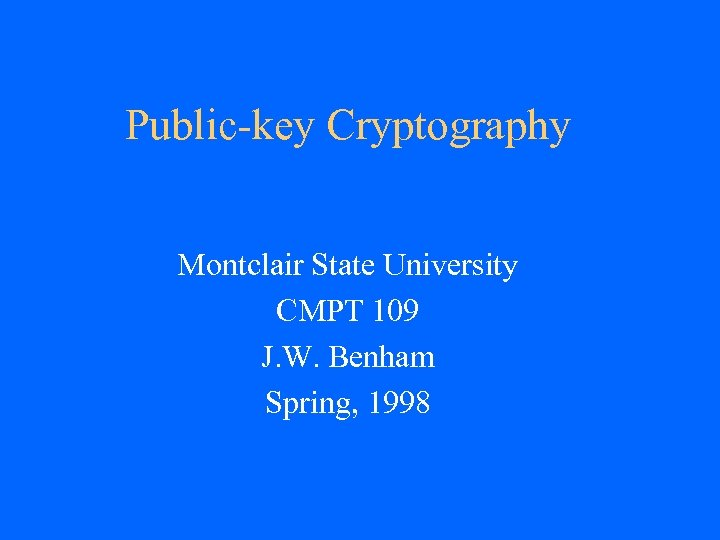Public-key Cryptography Montclair State University CMPT 109 J. W. Benham Spring, 1998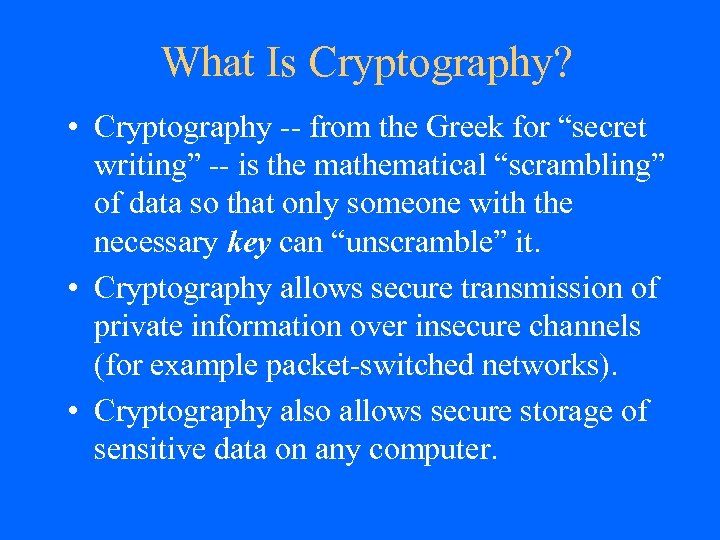What Is Cryptography? • Cryptography -- from the Greek for “secret writing” -- is the mathematical “scrambling” of data so that only someone with the necessary key can “unscramble” it. • Cryptography allows secure transmission of private information over insecure channels (for example packet-switched networks). • Cryptography also allows secure storage of sensitive data on any computer.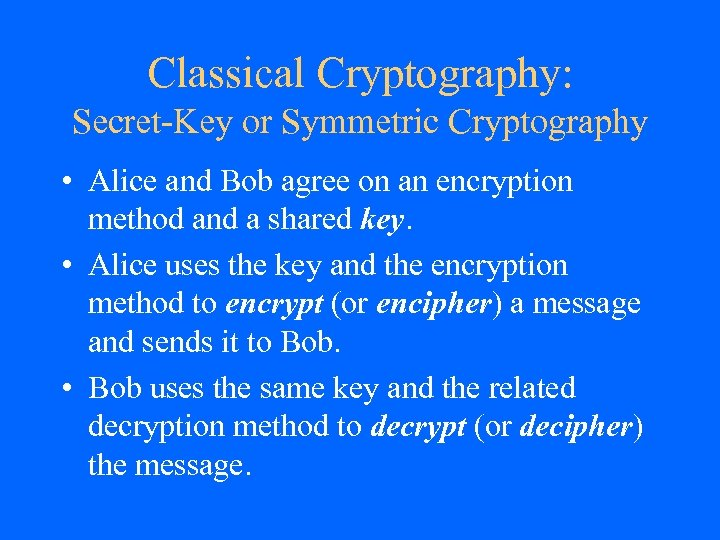Classical Cryptography: Secret-Key or Symmetric Cryptography • Alice and Bob agree on an encryption method and a shared key. • Alice uses the key and the encryption method to encrypt (or encipher) a message and sends it to Bob. • Bob uses the same key and the related decryption method to decrypt (or decipher) the message.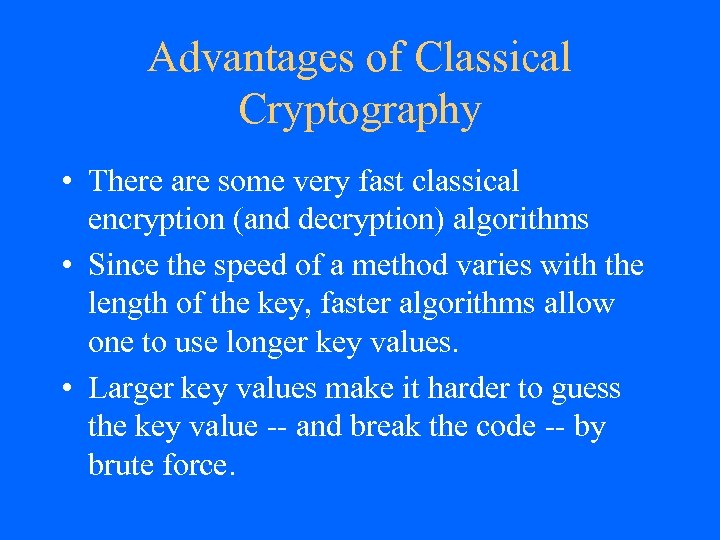Advantages of Classical Cryptography • There are some very fast classical encryption (and decryption) algorithms • Since the speed of a method varies with the length of the key, faster algorithms allow one to use longer key values. • Larger key values make it harder to guess the key value -- and break the code -- by brute force.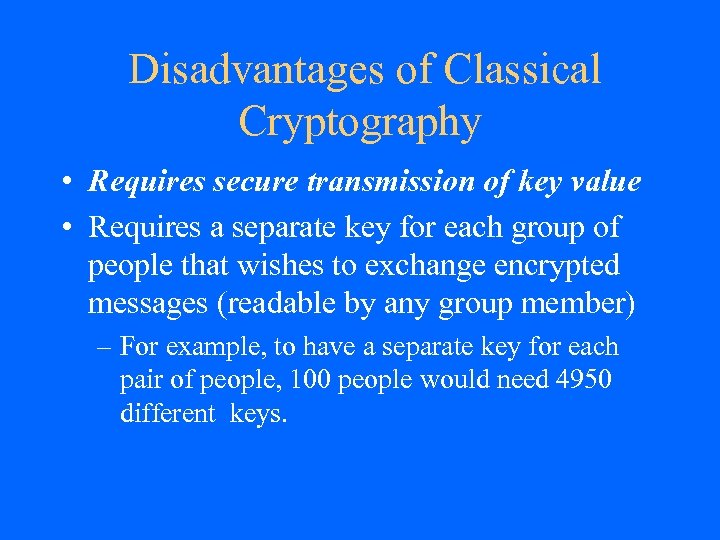Disadvantages of Classical Cryptography • Requires secure transmission of key value • Requires a separate key for each group of people that wishes to exchange encrypted messages (readable by any group member) – For example, to have a separate key for each pair of people, 100 people would need 4950 different keys.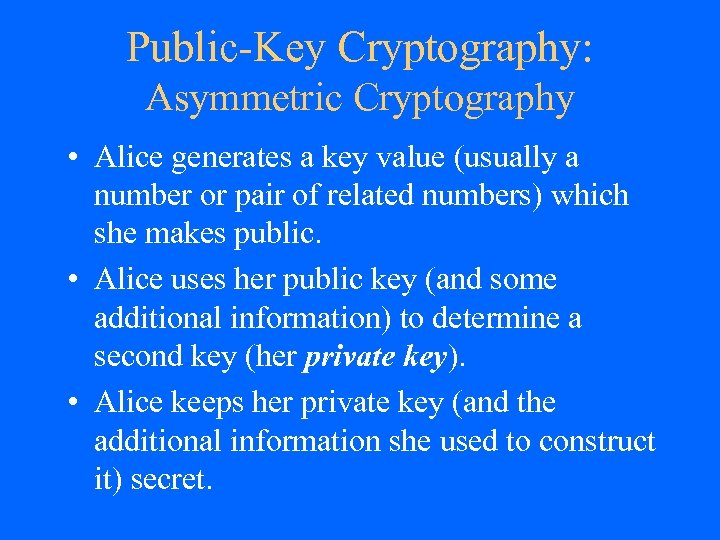Public-Key Cryptography: Asymmetric Cryptography • Alice generates a key value (usually a number or pair of related numbers) which she makes public. • Alice uses her public key (and some additional information) to determine a second key (her private key). • Alice keeps her private key (and the additional information she used to construct it) secret.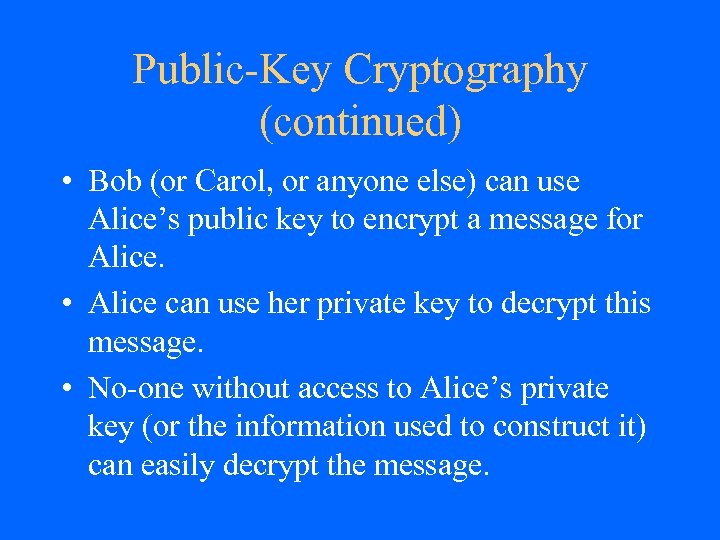Public-Key Cryptography (continued) • Bob (or Carol, or anyone else) can use Alice’s public key to encrypt a message for Alice. • Alice can use her private key to decrypt this message. • No-one without access to Alice’s private key (or the information used to construct it) can easily decrypt the message.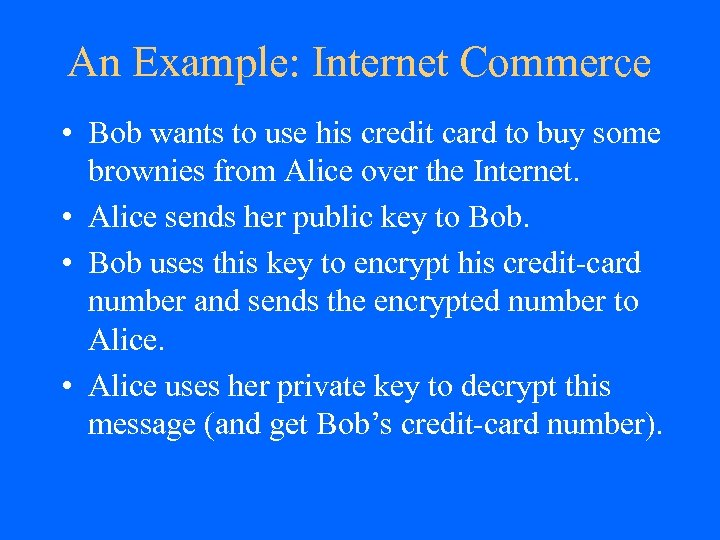An Example: Internet Commerce • Bob wants to use his credit card to buy some brownies from Alice over the Internet. • Alice sends her public key to Bob. • Bob uses this key to encrypt his credit-card number and sends the encrypted number to Alice. • Alice uses her private key to decrypt this message (and get Bob’s credit-card number).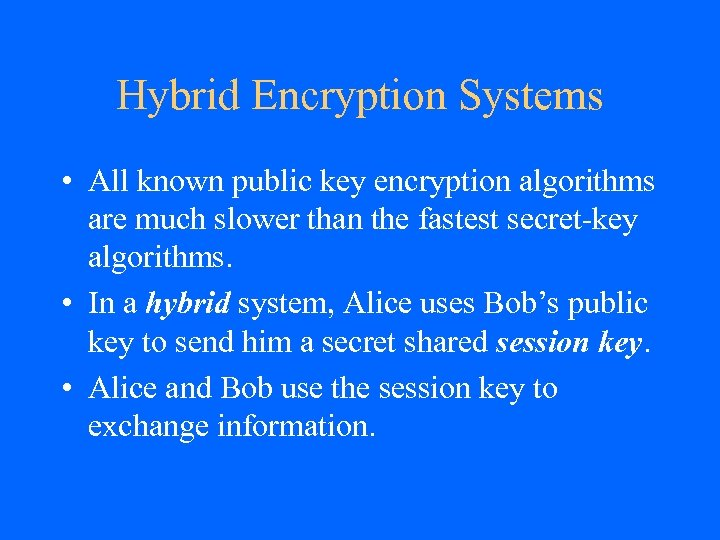Hybrid Encryption Systems • All known public key encryption algorithms are much slower than the fastest secret-key algorithms. • In a hybrid system, Alice uses Bob’s public key to send him a secret shared session key. • Alice and Bob use the session key to exchange information.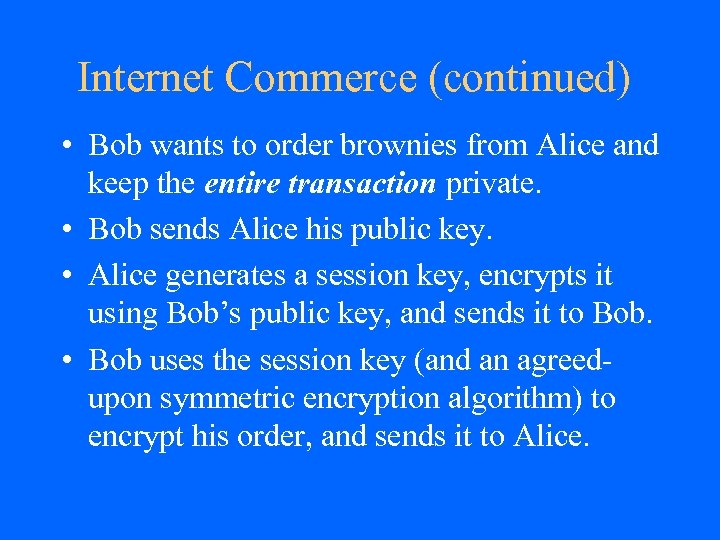Internet Commerce (continued) • Bob wants to order brownies from Alice and keep the entire transaction private. • Bob sends Alice his public key. • Alice generates a session key, encrypts it using Bob’s public key, and sends it to Bob. • Bob uses the session key (and an agreedupon symmetric encryption algorithm) to encrypt his order, and sends it to Alice.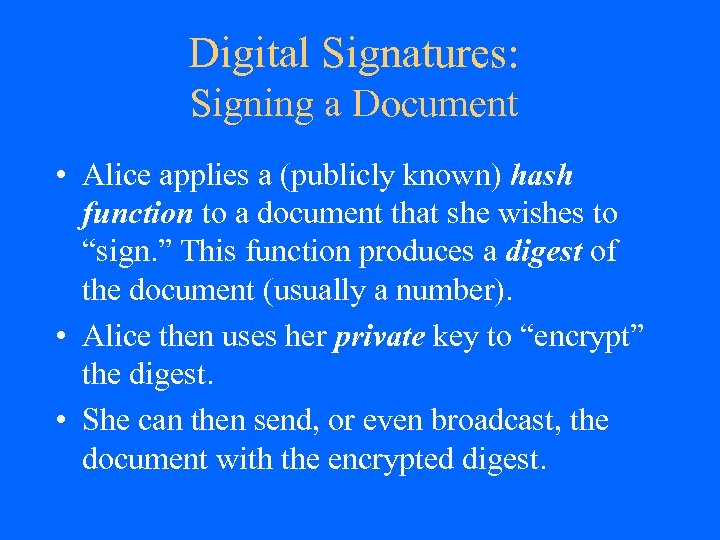Digital Signatures: Signing a Document • Alice applies a (publicly known) hash function to a document that she wishes to “sign. ” This function produces a digest of the document (usually a number). • Alice then uses her private key to “encrypt” the digest. • She can then send, or even broadcast, the document with the encrypted digest.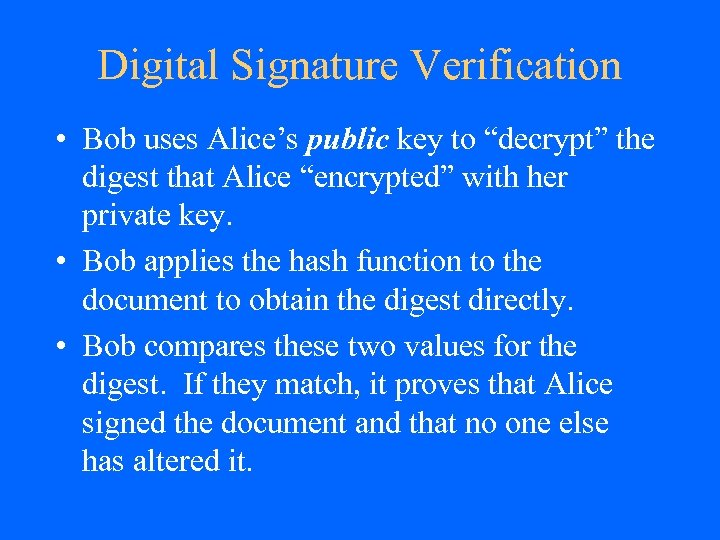Digital Signature Verification • Bob uses Alice’s public key to “decrypt” the digest that Alice “encrypted” with her private key. • Bob applies the hash function to the document to obtain the digest directly. • Bob compares these two values for the digest. If they match, it proves that Alice signed the document and that no one else has altered it.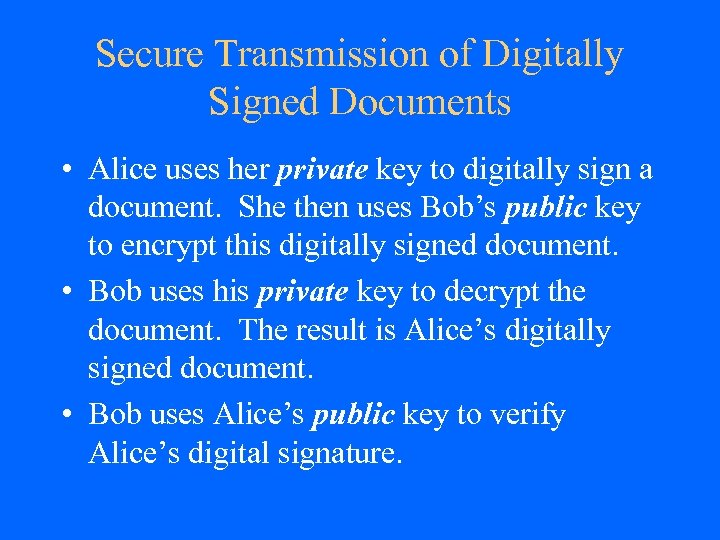Secure Transmission of Digitally Signed Documents • Alice uses her private key to digitally sign a document. She then uses Bob’s public key to encrypt this digitally signed document. • Bob uses his private key to decrypt the document. The result is Alice’s digitally signed document. • Bob uses Alice’s public key to verify Alice’s digital signature.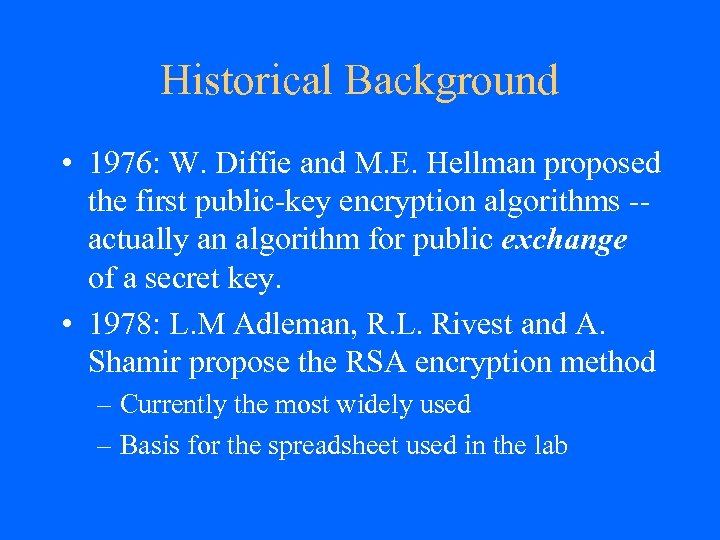Historical Background • 1976: W. Diffie and M. E. Hellman proposed the first public-key encryption algorithms -actually an algorithm for public exchange of a secret key. • 1978: L. M Adleman, R. L. Rivest and A. Shamir propose the RSA encryption method – Currently the most widely used – Basis for the spreadsheet used in the lab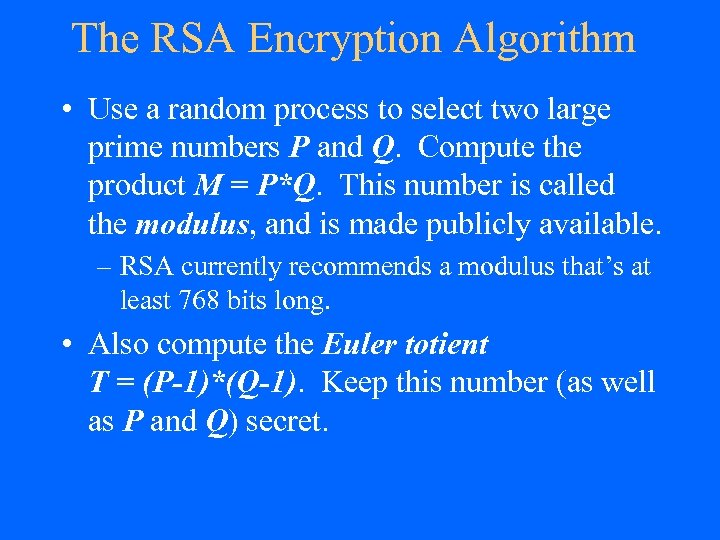The RSA Encryption Algorithm • Use a random process to select two large prime numbers P and Q. Compute the product M = P*Q. This number is called the modulus, and is made publicly available. – RSA currently recommends a modulus that’s at least 768 bits long. • Also compute the Euler totient T = (P-1)*(Q-1). Keep this number (as well as P and Q) secret.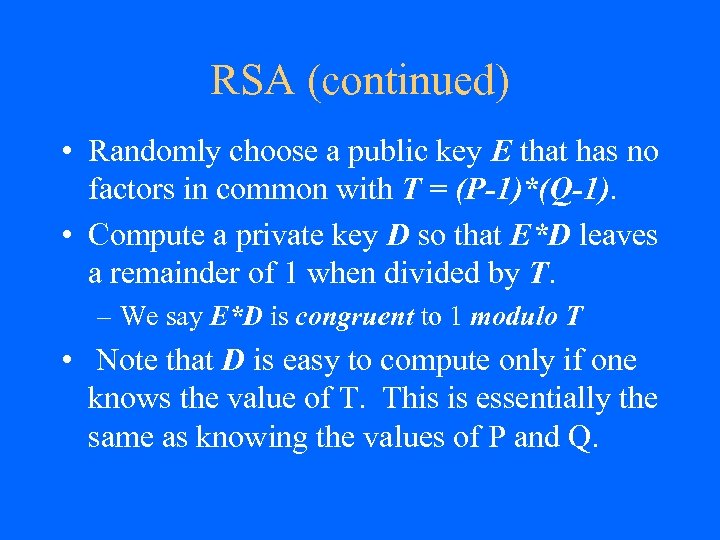RSA (continued) • Randomly choose a public key E that has no factors in common with T = (P-1)*(Q-1). • Compute a private key D so that E*D leaves a remainder of 1 when divided by T. – We say E*D is congruent to 1 modulo T • Note that D is easy to compute only if one knows the value of T. This is essentially the same as knowing the values of P and Q.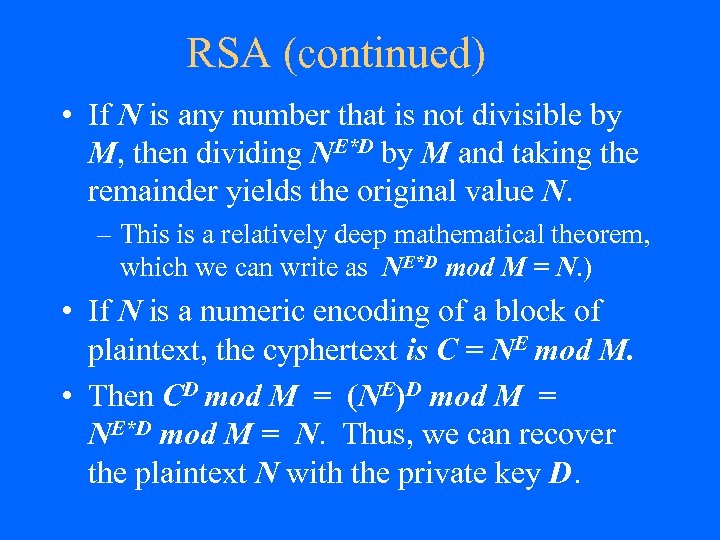RSA (continued) • If N is any number that is not divisible by M, then dividing NE*D by M and taking the remainder yields the original value N. – This is a relatively deep mathematical theorem, which we can write as NE*D mod M = N. ) • If N is a numeric encoding of a block of plaintext, the cyphertext is C = NE mod M. • Then CD mod M = (NE)D mod M = NE*D mod M = N. Thus, we can recover the plaintext N with the private key D.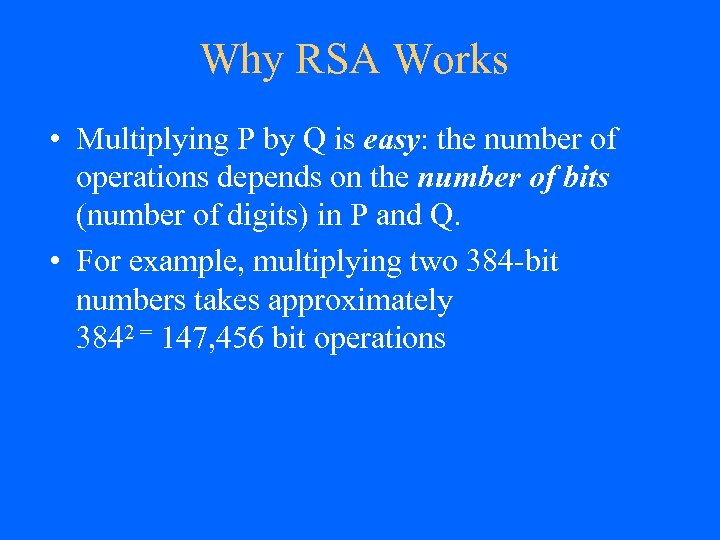Why RSA Works • Multiplying P by Q is easy: the number of operations depends on the number of bits (number of digits) in P and Q. • For example, multiplying two 384 -bit numbers takes approximately 3842 = 147, 456 bit operations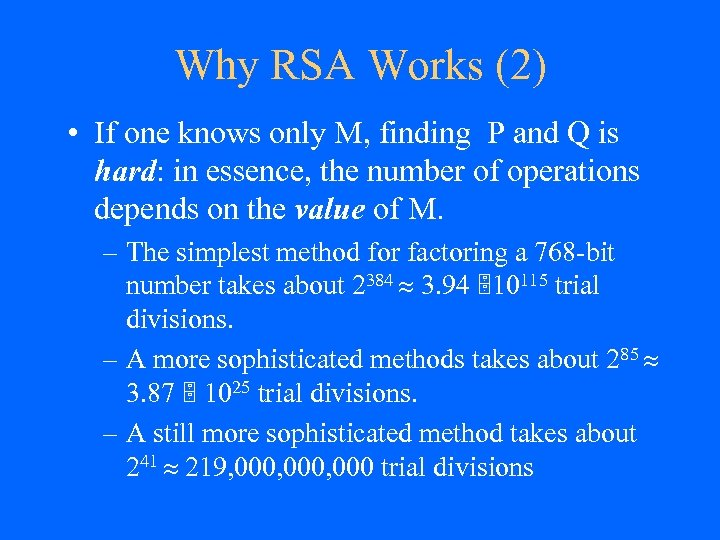Why RSA Works (2) • If one knows only M, finding P and Q is hard: in essence, the number of operations depends on the value of M. – The simplest method for factoring a 768 -bit number takes about 2384 3. 94 10115 trial divisions. – A more sophisticated methods takes about 285 3. 87 1025 trial divisions. – A still more sophisticated method takes about 241 219, 000, 000 trial divisions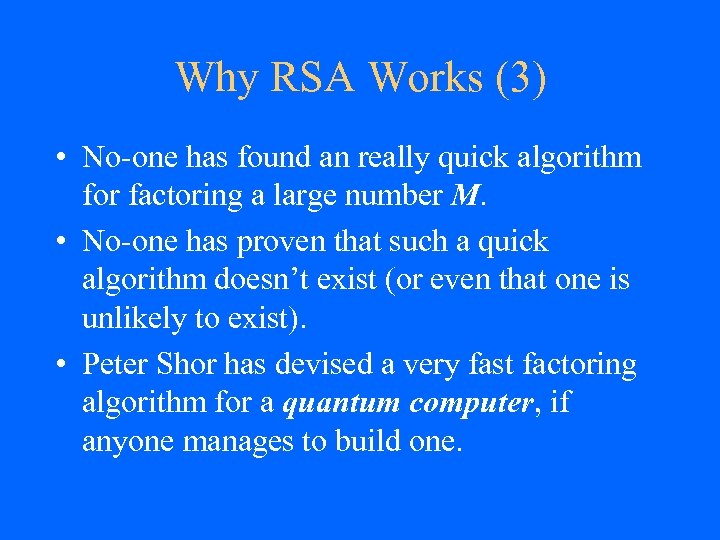Why RSA Works (3) • No-one has found an really quick algorithm for factoring a large number M. • No-one has proven that such a quick algorithm doesn’t exist (or even that one is unlikely to exist). • Peter Shor has devised a very fast factoring algorithm for a quantum computer, if anyone manages to build one.